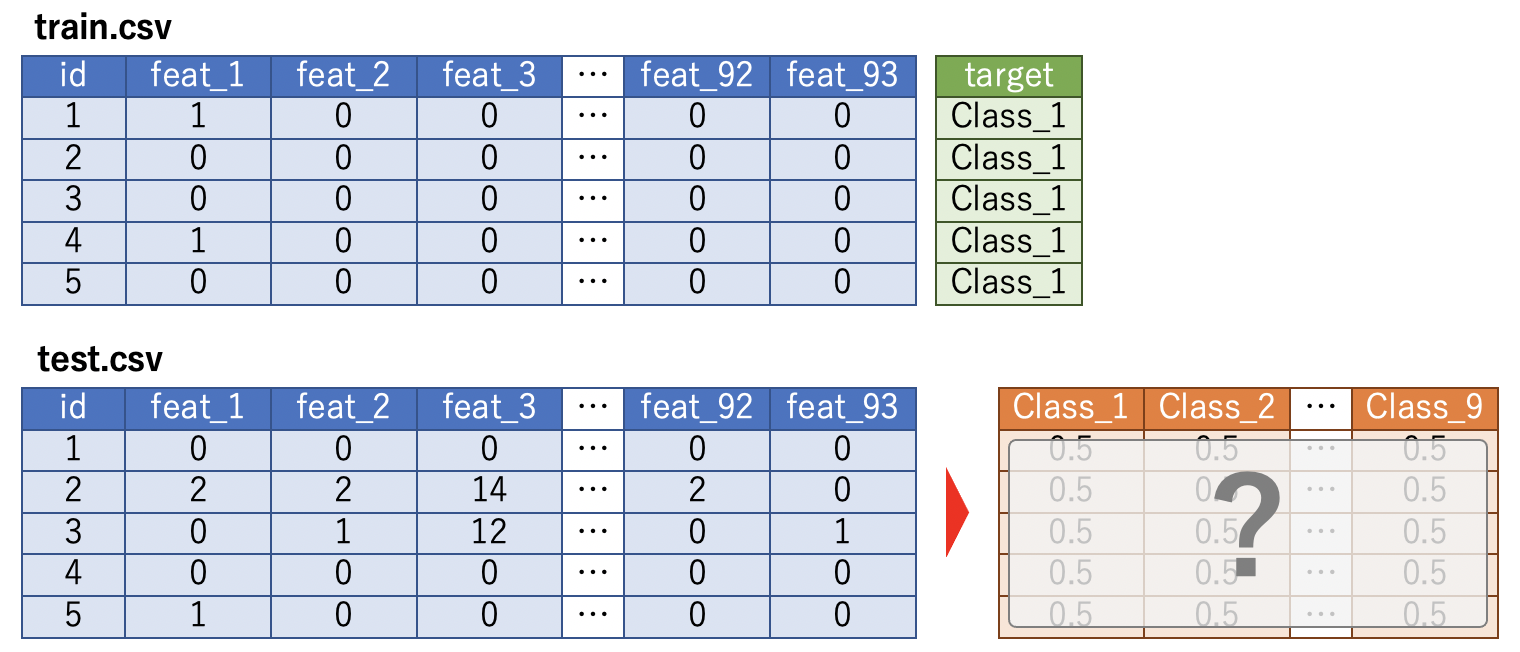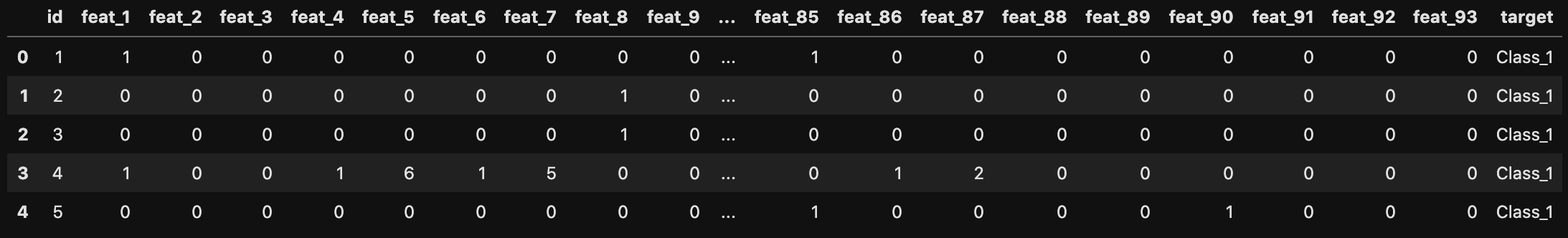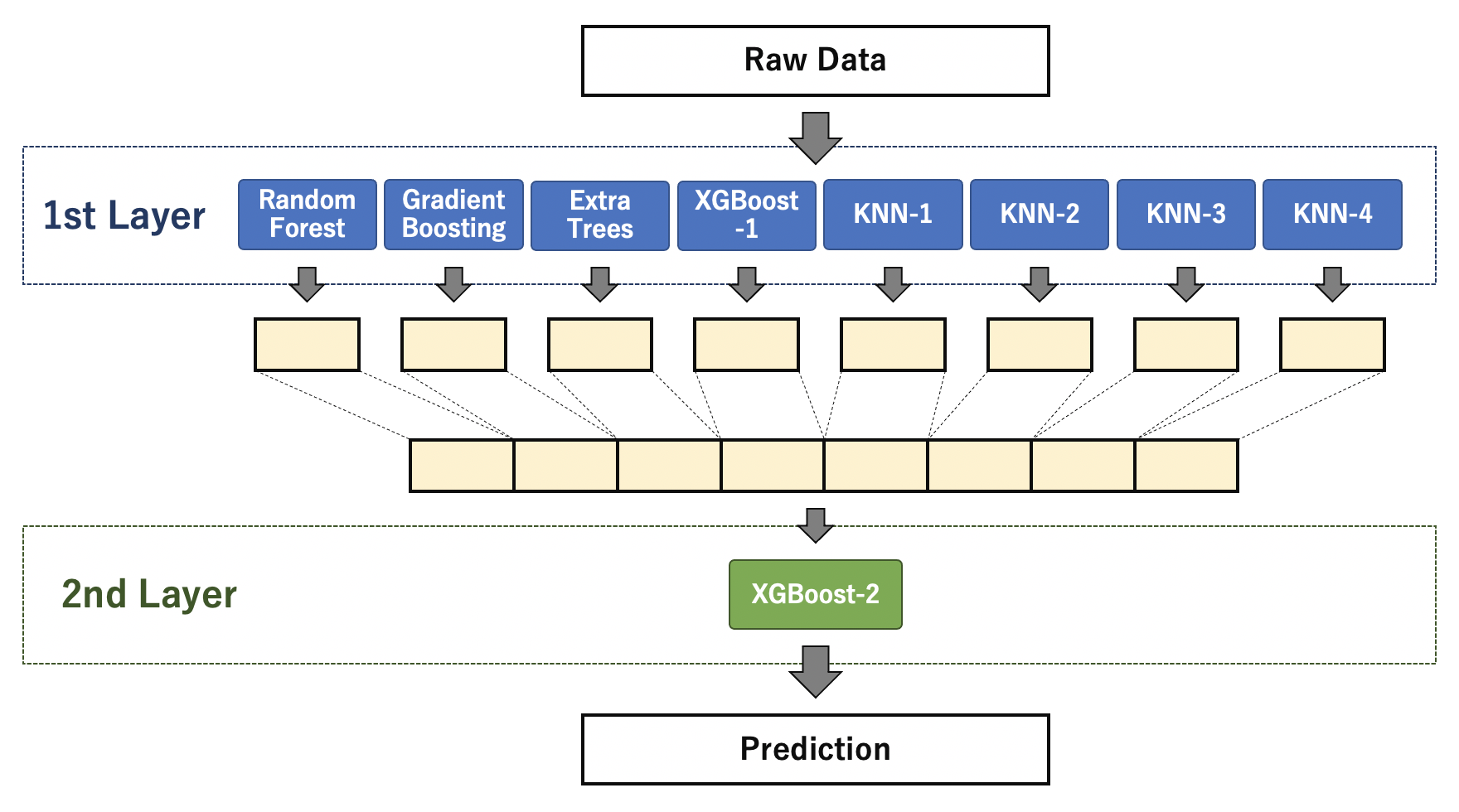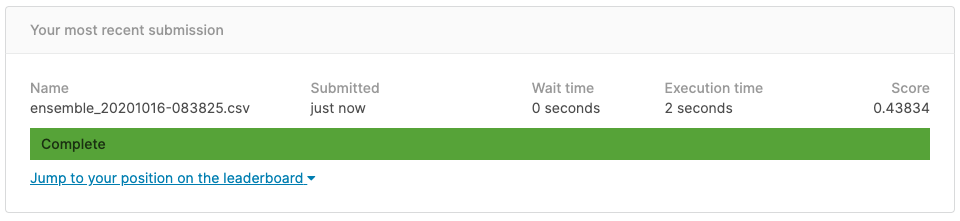# Implement stacking learning in Python [Kaggle]

TL;DR

Stacking learning is a commonly used method when the accuracy of a single prediction model in machine learning reaches a plateau. In this article, we will use Python to create a stacking model based on the past Kaggle competition "Otto Group Product Classification Challenge". Implement and challenge multiclass classification tasks.

## Competition overview

A multi-classification task that predicts which of the nine classes the product data will fall into. `train.csv` stores 93 features and the data of the class to which it belongs, which is the objective variable. The purpose is to predict the class to which each product belongs with probability from the features of `test.csv`. Multi-Class Log-Loss is used as the evaluation index.# Preparation

Import the required libraries.

#### `In`

``````
import os, sys
import datetime
import warnings
warnings.filterwarnings('ignore')

import pandas as pd
import numpy as np
import matplotlib.pyplot as plt
import seaborn as sns

from sklearn.model_selection import StratifiedKFold
from sklearn.preprocessing import LabelEncoder
from sklearn.ensemble import RandomForestClassifier
from sklearn.ensemble import ExtraTreesClassifier
from sklearn.neighbors import KNeighborsClassifier

import xgboost as xgb
from xgboost import XGBClassifier
``````

#### `In`

``````
``````

#### `In`

``````
``````Since the value of the objective variable is a character string, convert it to a numerical value.

#### `In`

``````
le = LabelEncoder()
le.fit(train['target'])
train['target'] = le.transform(train['target'])
``````

Separate the explanatory variable X and the objective variable y. Convert X to a NumPy array.

#### `In`

``````
X_train = train.drop(['id', 'target'], axis=1)
y_train = train['target'].copy()
X_test = test.drop(['id'], axis=1)

X_train = X_train.values
X_test = X_test.values
``````

Keep the `test` ʻid` field for creating the submission file.

``````testIds = test['id'].copy()
``````

Since it deviates from the purpose, I will omit it here, but when looking at the distribution of the data, there is a considerable bias in the values. I thought that normalization was a good method, but when I tried it, there was no improvement in the final accuracy, so I decided to proceed with Row Data as it is.

# Definition of the first layer model

## Overall model configuration

As an overall configuration, define eight models such as Random Forest, Gradient Boosting, and KNN in the first layer. Using the predicted values of each model in the first layer, the prediction by XGBoost in the second layer is used as the final prediction result.## Definition of classifier extension class

Define an extension class for the classifier to simplify operations (definition, training, prediction) for each first-tier model.

#### `In`

``````
class ClfBuilder(object):
def __init__(self, clf, params=None):
self.clf = clf(**params)

def fit(self, X, y):
self.clf.fit(X, y)

def predict(self, X):
return self.clf.predict(X)

def predict_proba(self, X):
return self.clf.predict_proba(X)
``````

## Definition of Out-of-Fold Prediction Function

Stacking uses the predicted values of the first layer model for the second layer model. In order to prevent overfitting of known data in the second layer, the predicted value by Out-of-Fold is calculated in the first layer and used for training in the second layer. In the following implementation, `Stratified KFold` is used for 5-fold cross-validation.

#### `In`

``````
def get_base_model_preds(clf, X_train, y_train, X_test):
print(clf.clf)

N_SPLITS = 5
oof_valid = np.zeros((X_train.shape, 9))
oof_test = np.zeros((X_test.shape, 9))
oof_test_skf = np.zeros((N_SPLITS, X_test.shape, 9))

skf = StratifiedKFold(n_splits=N_SPLITS)
for i, (train_index, valid_index) in enumerate(skf.split(X_train, y_train)):
print('[CV] {}/{}'.format(i+1, N_SPLITS))
X_train_, X_valid_ = X_train[train_index], X_train[valid_index]
y_train_, y_valid_ = y_train[train_index], y_train[valid_index]

clf.fit(X_train_, y_train_)

oof_valid[valid_index] = clf.predict_proba(X_valid_)
oof_test_skf[i, :] = clf.predict_proba(X_test)

oof_test[:] = oof_test_skf.mean(axis=0)
return oof_valid, oof_test
``````

## Parameter setting

Set the parameter to be passed to the `ClfBuilder` function with dict type. (* Hyperparameter tuning is not performed here)

#### `In`

``````
rfc_params = {
'n_estimators': 100,
'max_depth': 10,
'random_state': 0,
}
gbc_params = {
'n_estimators': 50,
'max_depth': 10,
'random_state': 0,
}
etc_params = {
'n_estimators': 100,
'max_depth': 10,
'random_state': 0,
}
xgbc1_params = {
'n_estimators': 100,
'max_depth': 10,
'random_state': 0,
}
knn1_params = {'n_neighbors': 4}
knn2_params = {'n_neighbors': 8}
knn3_params = {'n_neighbors': 16}
knn4_params = {'n_neighbors': 32}
``````

Create an instance of the first layer model.

#### `In`

``````
rfc = ClfBuilder(clf=RandomForestClassifier, params=rfc_params)
etc = ClfBuilder(clf=ExtraTreesClassifier, params=etc_params)
xgbc1 = ClfBuilder(clf=XGBClassifier, params=xgbc1_params)
knn1 = ClfBuilder(clf=KNeighborsClassifier, params=knn1_params)
knn2 = ClfBuilder(clf=KNeighborsClassifier, params=knn2_params)
knn3 = ClfBuilder(clf=KNeighborsClassifier, params=knn3_params)
knn4 = ClfBuilder(clf=KNeighborsClassifier, params=knn4_params)
``````

# Learning the first layer model

Using the `get_base_model_preds` defined earlier, each 1st layer model is trained and the predicted value used for training and prediction of the 2nd layer model is calculated.

#### `In`

``````
oof_valid_rfc, oof_test_rfc = get_base_model_preds(rfc, X_train, y_train, X_test)
oof_valid_gbc, oof_test_gbc = get_base_model_preds(gbc, X_train, y_train, X_test)
oof_valid_etc, oof_test_etc = get_base_model_preds(etc, X_train, y_train, X_test)
oof_valid_xgbc1, oof_test_xgbc1 = get_base_model_preds(xgbc1, X_train, y_train, X_test)
oof_valid_knn1, oof_test_knn1 = get_base_model_preds(knn1, X_train, y_train, X_test)
oof_valid_knn2, oof_test_knn2 = get_base_model_preds(knn2, X_train, y_train, X_test)
oof_valid_knn3, oof_test_knn3 = get_base_model_preds(knn3, X_train, y_train, X_test)
oof_valid_knn4, oof_test_knn4 = get_base_model_preds(knn4, X_train, y_train, X_test)
``````

#### `Out`

``````
RandomForestClassifier(max_depth=10, random_state=0)
[CV] 1/5
[CV] 2/5
[CV] 3/5
[CV] 4/5
[CV] 5/5
[CV] 1/5

(...Abbreviation...)

[CV] 5/5
KNeighborsClassifier(n_neighbors=32)
[CV] 1/5
[CV] 2/5
[CV] 3/5
[CV] 4/5
[CV] 5/5
``````

The prediction value to be input to the second layer is the combination of the prediction results of each classifier side by side.

#### `In`

``````
X_train_base = np.concatenate([oof_valid_rfc,
oof_valid_gbc,
oof_valid_etc,
oof_valid_xgbc1,
oof_valid_knn1,
oof_valid_knn2,
oof_valid_knn3,
oof_valid_knn4,
], axis=1)
X_test_base = np.concatenate([oof_test_rfc,
oof_test_gbc,
oof_test_etc,
oof_test_xgbc1,
oof_test_knn1,
oof_test_knn2,
oof_test_knn3,
oof_test_knn4,
], axis=1)
``````

# Definition / learning of the second layer model

XGBoost is used as the second layer model. Set the parameters and instantiate the model.

#### `In`

``````
xgbc2_params = {
'n_eetimators': 100,
'max_depth': 5,
'random_state': 42,
}
xgbc2 = XGBClassifier(**xgbc2_params)
``````

We will train the second layer model.

#### `In`

``````
xgbc2.fit(X_train_base, y_train)
``````

# Prediction by test data

Prediction is made using test data using the trained second-layer model.

#### `In`

``````
prediction = xgbc2.predict_proba(X_test_base)
``````

Store the prediction results in the data frame for the submission file. Output in csv format and submit.

#### `In`

``````
columns = ['Class_1', 'Class_2', 'Class_3', 'Class_4', 'Class_5', 'Class_6', 'Class_7', 'Class_8', 'Class_9']
df_prediction = pd.DataFrame(prediction, columns=columns)
df_submission = pd.concat([testIds, df_prediction], axis=1)
``````

#### `In`

``````
now = datetime.datetime.now()
timestamp = now.strftime('%Y%m%d-%H%M%S')
df_submission.to_csv('output/ensemble_{}.csv'.format(timestamp), index=False)
``````The result is `Score = 0.443834`. Since it is a late submission, it will not be listed on the Leaderboard, but if it is listed, it was ranked 462/3507, which was the top 14%.

## Accuracy comparison with each model on the first layer

To see the effect of stacking, let's compare it with the predicted score for the test data calculated by each model in the first layer.

Classifier Score
Random Forest 0.95957